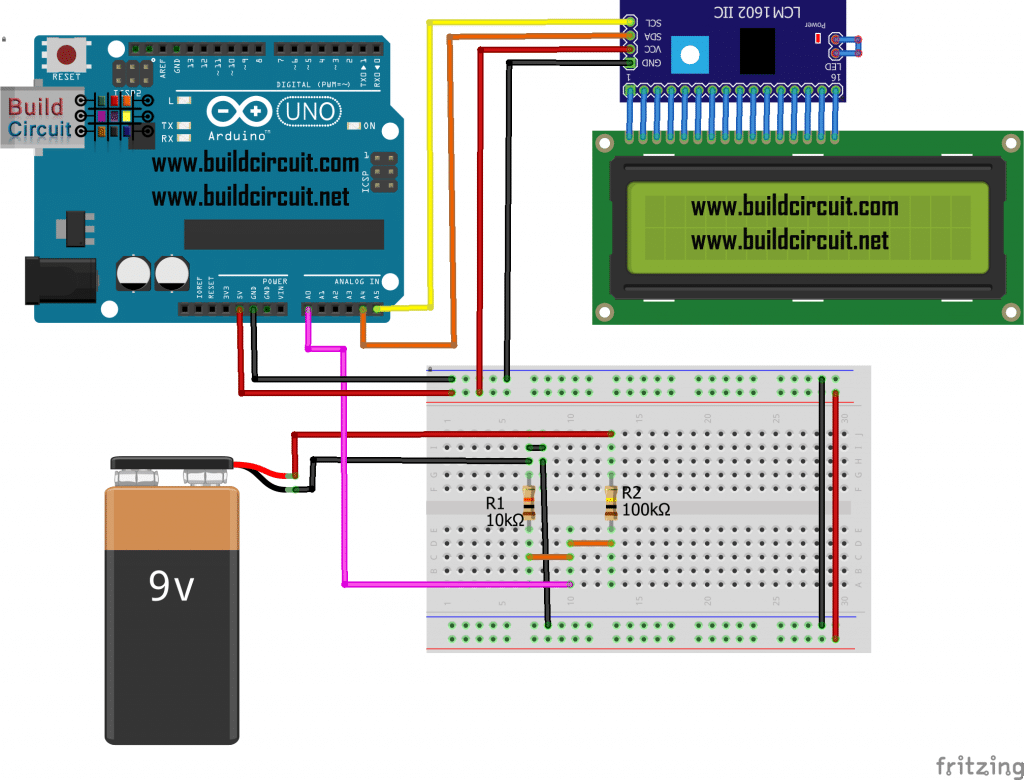In this tutorial,  we will build a circuit with Arduino Uno that can read voltages from 0 to 30V DC and display them on the 16×2 display, the ideal project to build our DIY voltmeter.

Thanks to the analogue inputs, that can be used to measure voltages from 0 to 5V. For higher values ​​we will use a voltage divider formed by 2 resistors in series, and we will measure the voltage of the central node connecting it to the analog input A0.Arduino converts the voltage detected on the analog Pin and converts it into digital format so that it can be processed by the microcontroller.
All that remains is to create the voltage divider with the two resistors and connect the display following the diagram below.

Sketch for I2C LCD:

16×2 LCD sketch